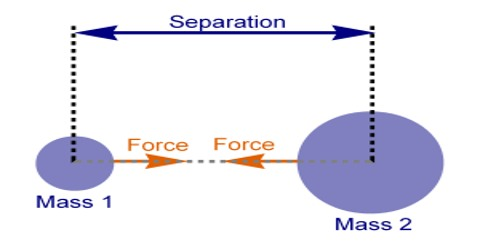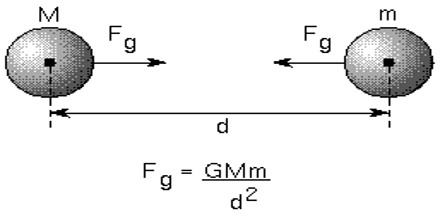Physics

# Explain Newton’s Law of GravitationNewton’s law of gravitation is stated below:

Statement: Any two particles in the universe attract each other with a force which is directly proportional to the product of their masses and inversely proportional to the square of the distance between them. This force acts along the line joining the two particles or objects.

This was discovered in 1687.

Explanation: By analyzing Newton’s law of gravitation it is seen that there are three segments of this law of which two are related to quantity of force and one is related to nature of force.

Let two particles of masses m1 and m2 be separated by a distance d [Figure]. If the force between them is F, then according to Newton’s law of gravitation-(i) F ∞ m1m2 (when d is constant)

and (ii) F ∞ 1/d2 (when m1 and m2 are constants)

Combining (i) and (ii), we get,

F ∞ m1m2 / d2 (when m1, m2 and d are vary)

or, F = G (m1m2 / d2)

where G is a constant of proportionality and is known as universal gravitational constant. This constant is called universal constant, because it does not depend on the nature of medium between the particles, nor does it depend on permeability, susceptibility, directivity and physical conditions of the particles.